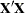The REG Procedure
 Computational Methods

The REG procedure first composes a crossproducts matrix. The matrix can be calculated from input data, reformed from an input correlation matrix, or read in from an SSCP data set. For each model, the procedure selects the appropriate crossproducts from the main matrix. The normal equations formed from the crossproducts are solved by using a sweep algorithm (Goodnight 1979). The method is accurate for data that are reasonably scaled and not too collinear.

The mechanism that PROC REG uses to check for singularity involves the diagonal (pivot) elements ofas it is being swept. If a pivot is less than SINGULAR*CSS, then a singularity is declared and the pivot is not swept (where CSS is the corrected sum of squares for the regressor and SINGULAR is machine dependent but is approximately 1E7 on most machines or reset in the PROC REG statement).

The sweep algorithm is also used in many places in the model-selection methods. The RSQUARE method uses the leaps-and-bounds algorithm by Furnival and Wilson (1974).Previous Page | Next Page | Top of Page